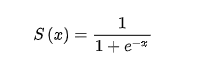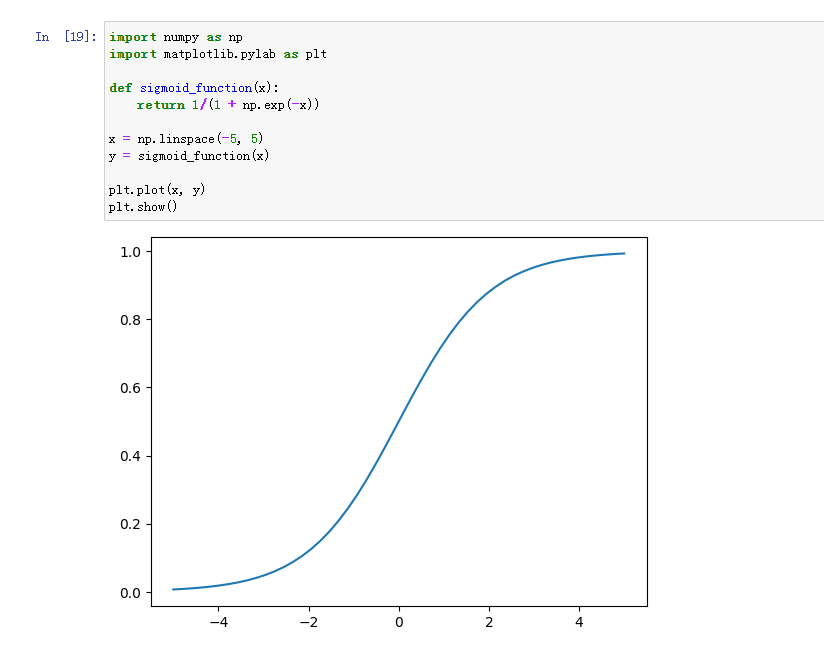﻿ Python求sigmoid函数 – 蒋智昊的博客# Python求sigmoid函数sigmoid函数也叫Logistic函数，用于隐层神经元输出，取值范围为(0,1)，它可以将一个实数映射到(0,1)的区间，可以用来做二分类。```import numpy as np
import matplotlib.pylab as plt

def sigmoid_function(x):
return 1/(1 + np.exp(-x))

x = np.linspace(-5, 5)
y = sigmoid_function(x)

plt.plot(x, y)
plt.show()```

np.exp(x)表示自然数e(2.718281828459045)的多少次方,括号里给数字1,就表示e的1次方,给2表示e的2次方,括号里的数可以是负数或分数。